views 1160 words

## 基础模型（Basic Models）

[Sutskever et al., 2014. Sequence to sequence learning with neural networks]

[Cho et al., 2014. Learning phrase representations using RNN encoder-decoder for statistical machine translation]

Sequence to sequence (seq2seq)模型在机器翻译和语音识别方面都有着广泛的应用.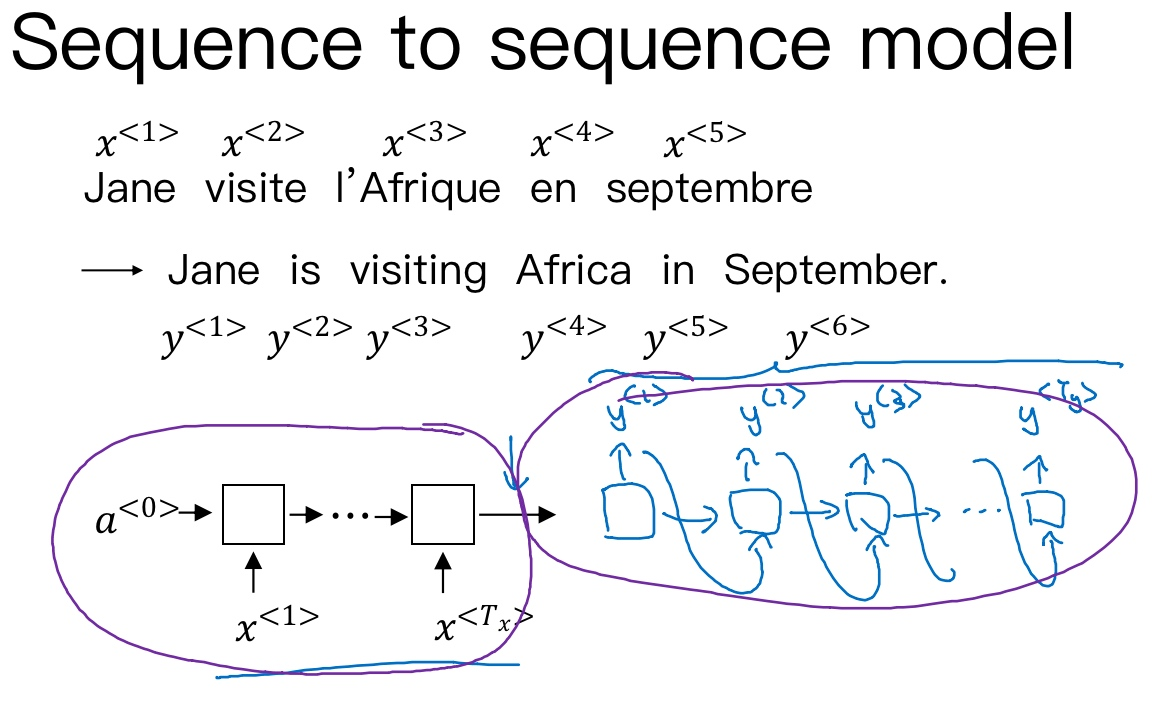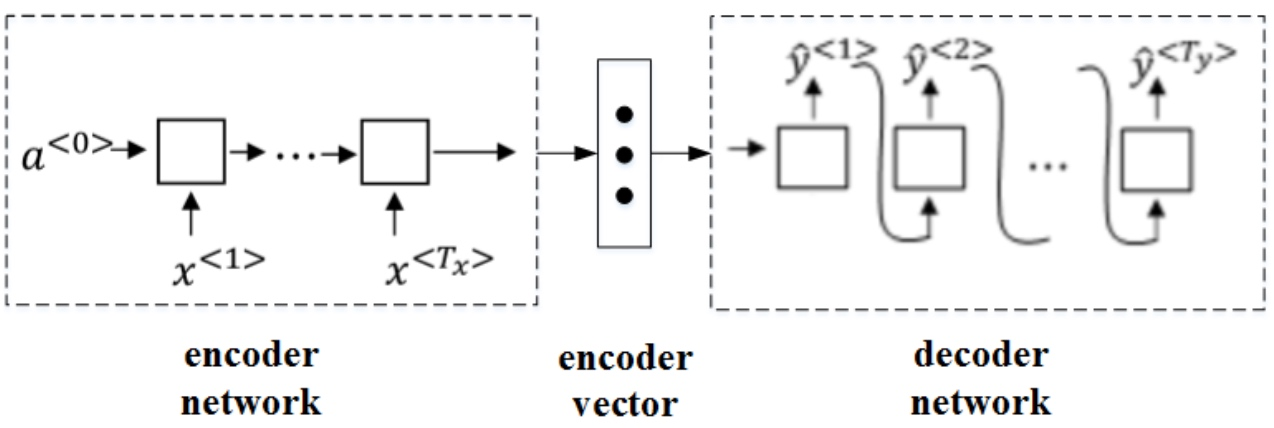[Mao et. al., 2014. Deep captioning with multimodal recurrent neural networks]

[Vinyals et. al., 2014. Show and tell: Neural image caption generator]

[Karpathy and Li, 2015. Deep visual-semantic alignments for generating image descriptions]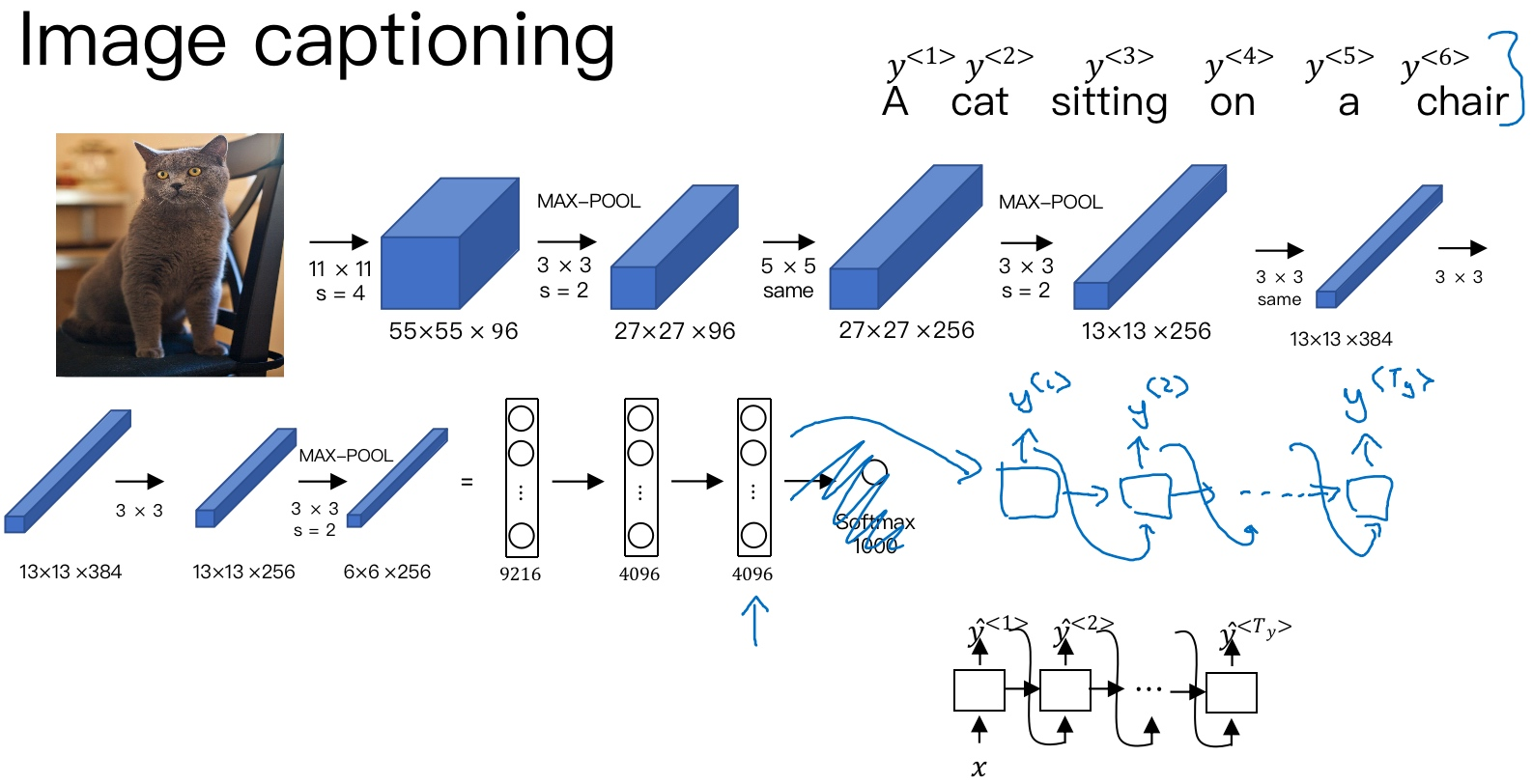## 选择最可能的句子（Picking the most likely sentence）

Sequence to sequence machine translation模型与language模型有一些相似，但也存在不同之处. 二者模型结构如下所示：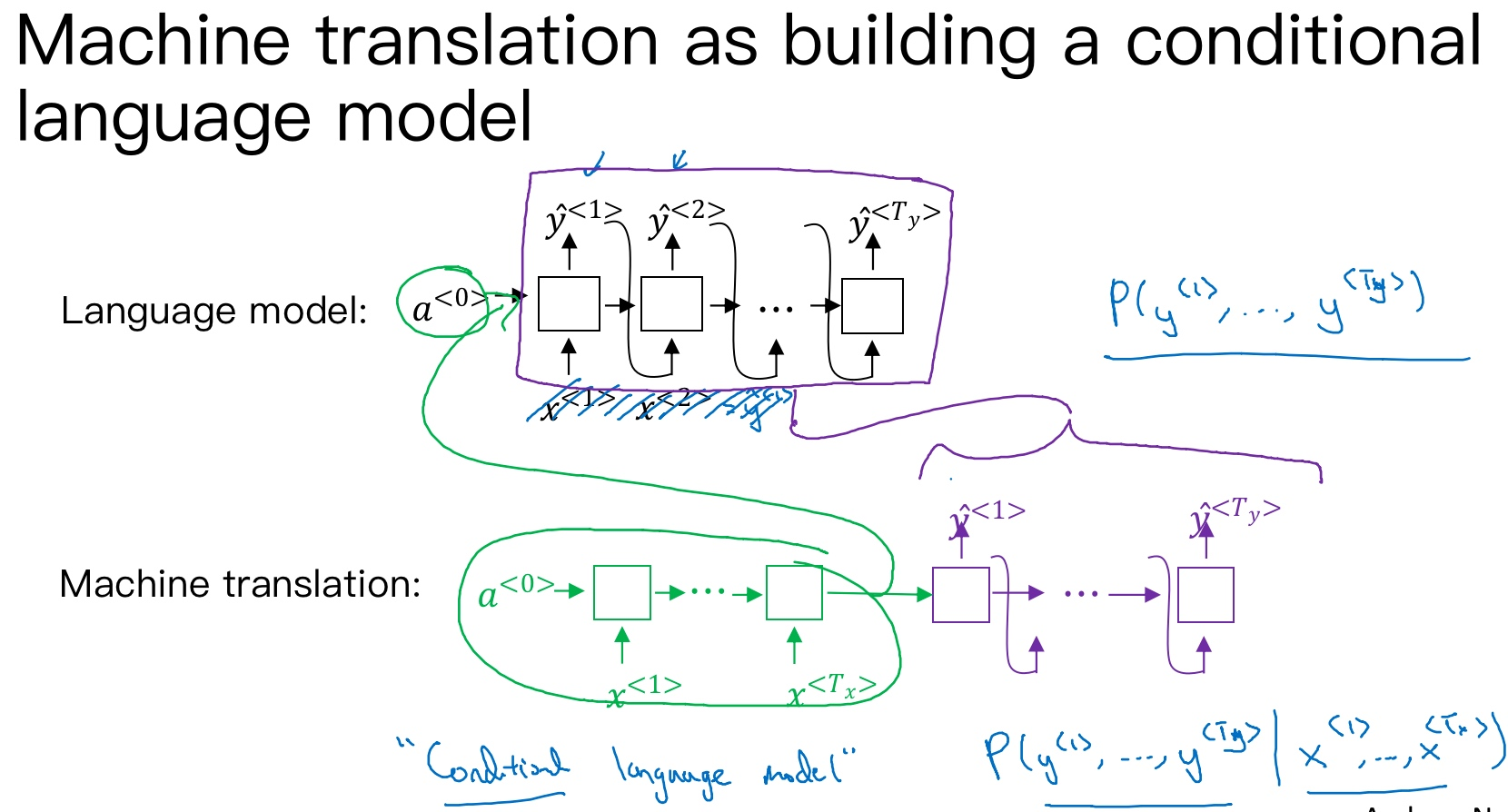• Language model是自动生成一条完整语句，语句是随机的
• 而machine translation model是根据输入语句，进行翻译，生成另外一条完整语句
• 上图中，绿色部分表示encoder network，紫色部分表示decoder network.
• decoder network与language model是相似的，encoder network可以看成是language model的$a^{<0>}$
• 语言模型总是以零向量开始，而encoder网络会计算出一系列向量来表示输入的句子
• decoder网络就可以以这个句子开始, 相当于它是模型的一个条件. 也就是说，在输入语句的条件下，生成正确的翻译语句.
• 因此，machine translation可以看成是有条件的语言模型(conditional language model). 这就是二者之间的区别与联系

$max\ P(y^{<1>},y^{<2>},\cdots,y^{<T_y>}|x^{<1>},x^{<2>},\cdots,x^{<T_x>})$

$$\mathop{argmax}_{y^{<1>},...,y^{<T_y>}}{P(y^{\lt 1 \gt},\cdots,y^{\lt T_y \gt}|x)}$$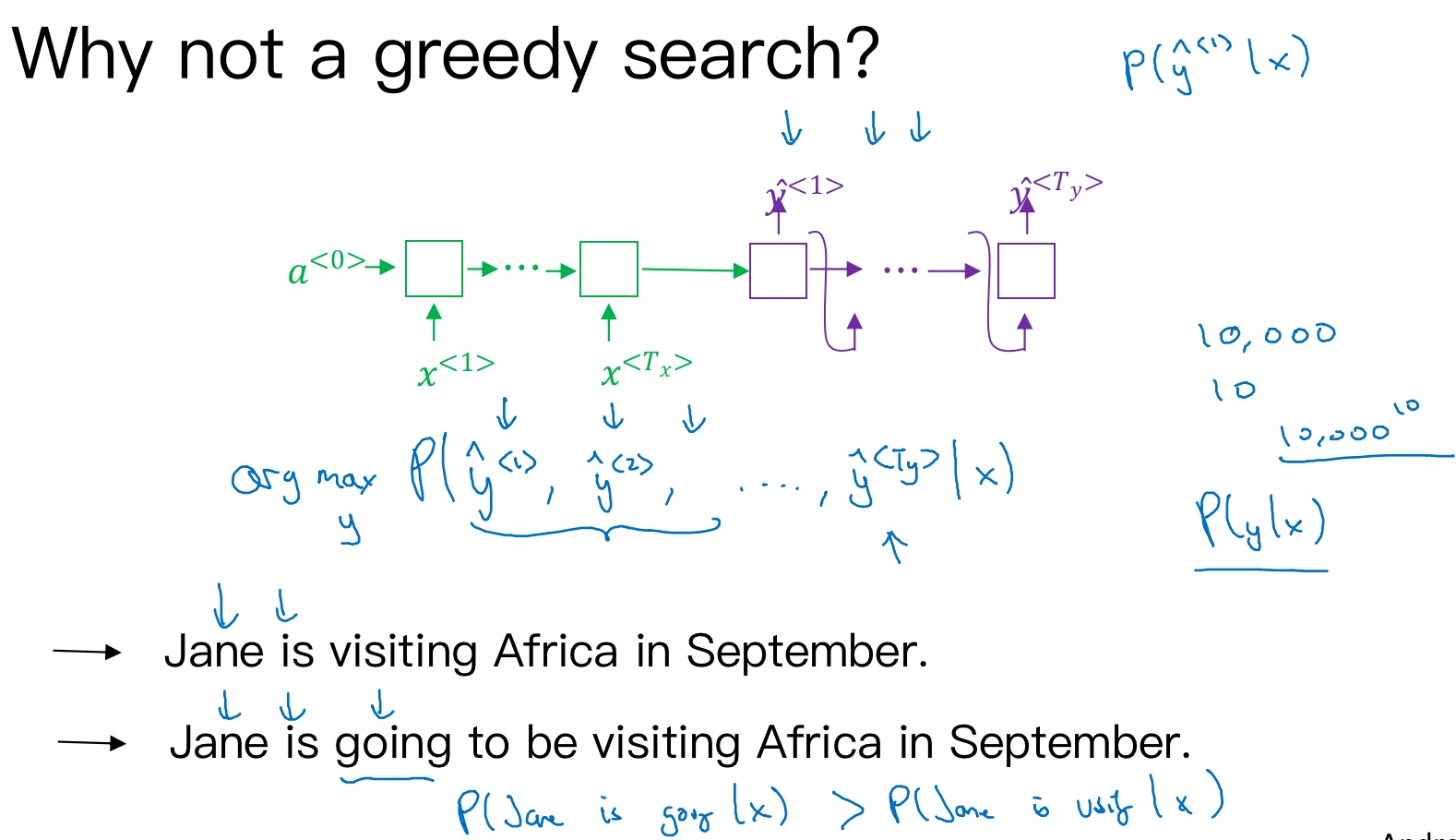Greedy search存在一些缺点.

1. 首先，因为greedy search每次只搜索一个单词，没有考虑该单词前后关系，概率选择上有可能会出错. 例如，上面翻译语句中，第三个单词’going’比’visiting’更常见，模型很可能会错误地选择了’going’, 而错失最佳翻译语句.
2. 其次，greedy search一次仅仅挑选一个词, 大大增加了运算成本，降低运算速度. 如果字典中有10,000个单词(即每个softmax output都是10,000)，并且翻译可能有10个词，那么可能的组合就有10,000的10次方

Greedy search每次是找出预测概率最大一个的单词，而beam search则是每次找出预测概率最大的B个单词. 集束搜索算法会有一个参数B表示取概率最大的单词个数(beam width), 可调. 本例中，令B=3, 表示一次会考虑3个结果.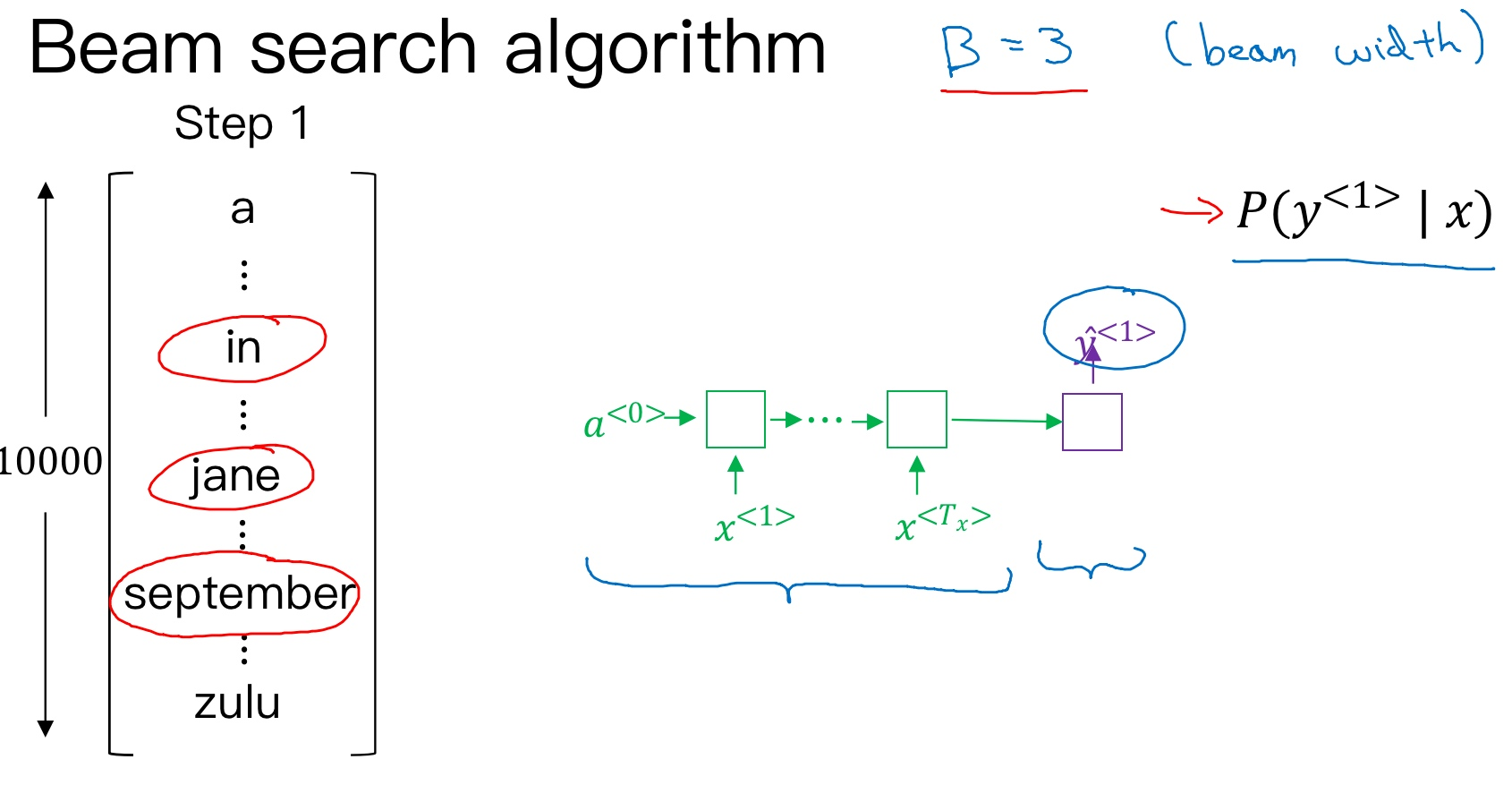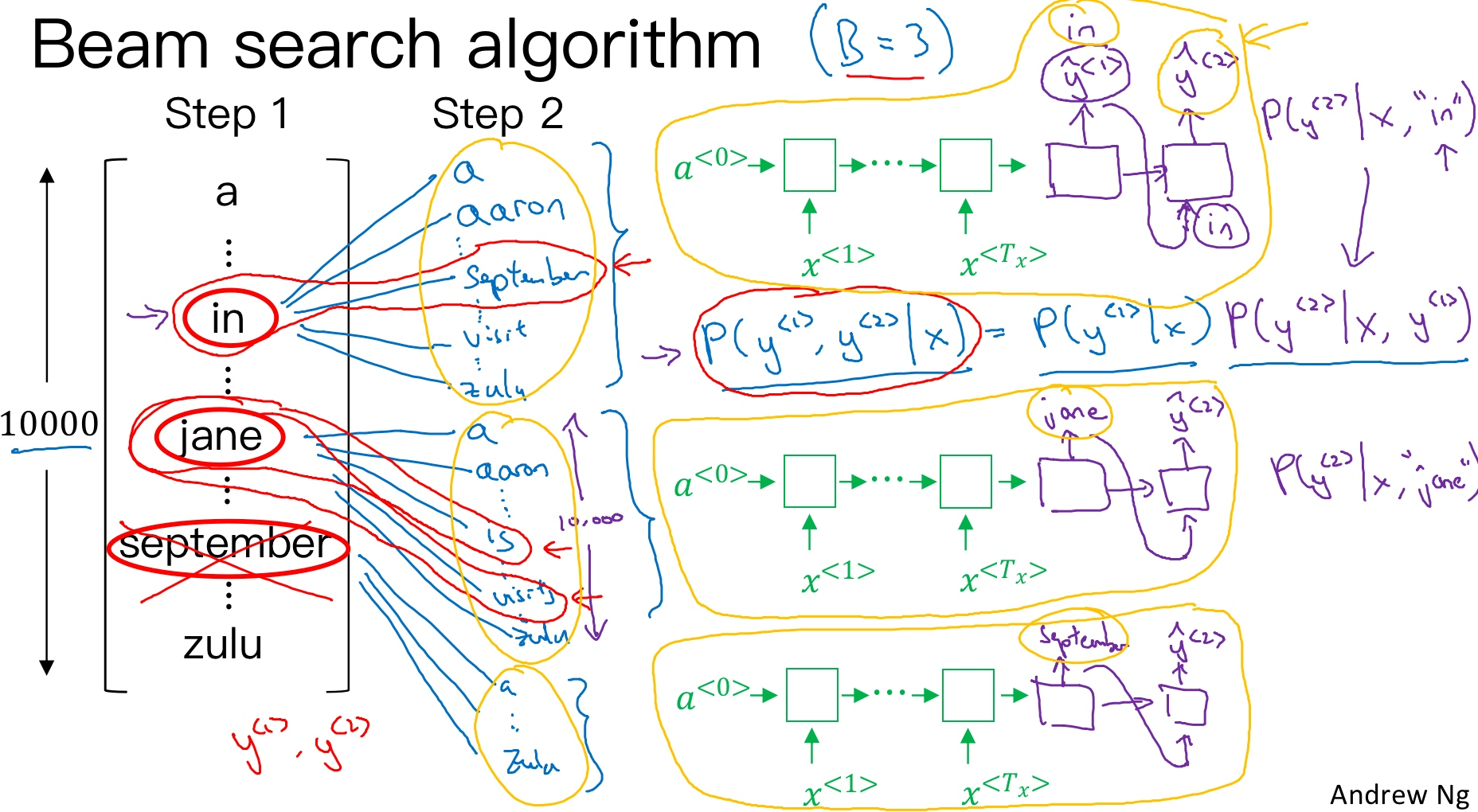$P(\hat y^{<1>},\hat y^{<2>}|x)=P(\hat y^{<1>} | x)\cdot P(\hat y^{<2>}|x,\hat y^{<1>})$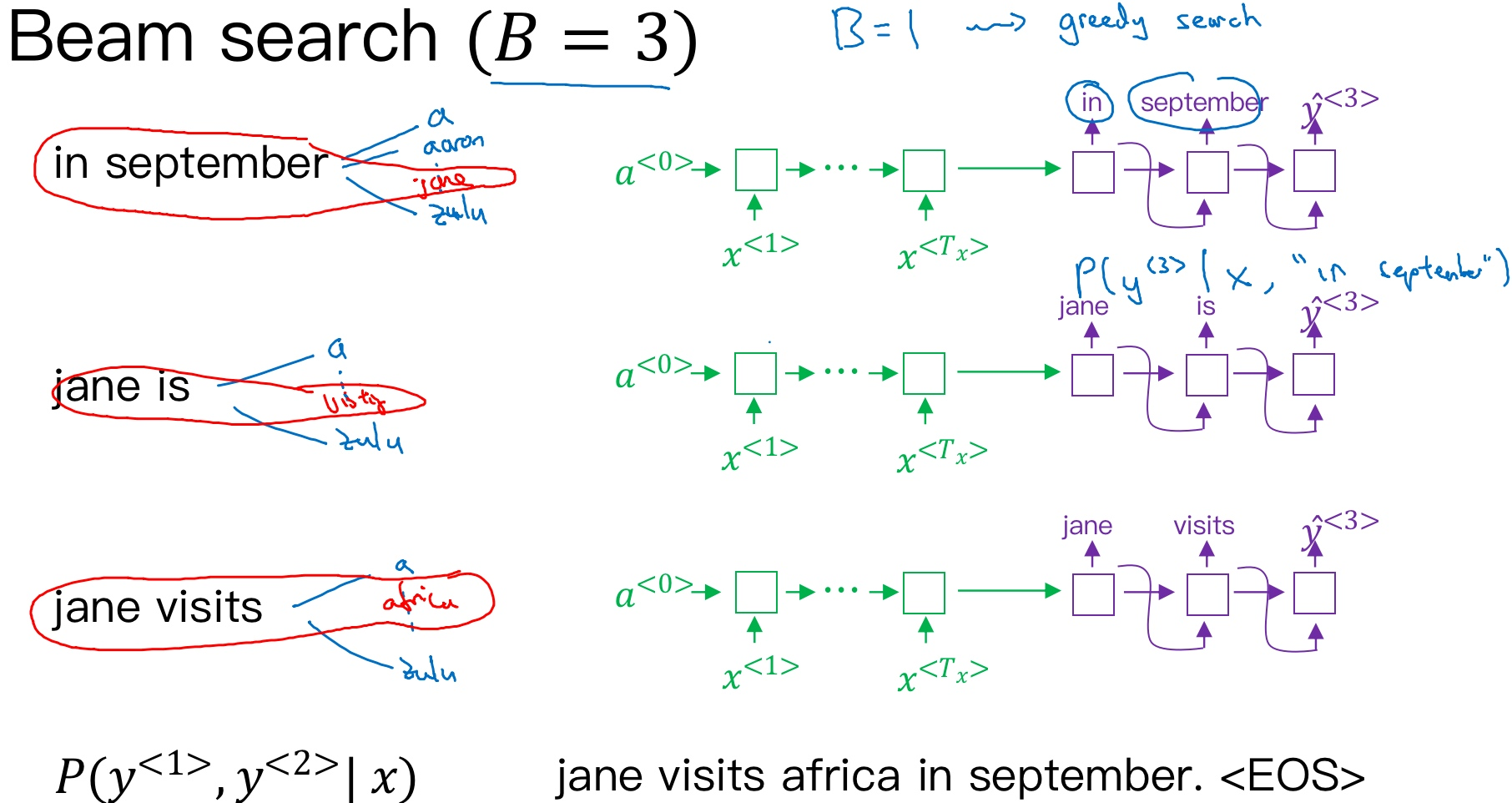$P(\hat y^{<1>},\hat y^{<2>},\hat y^{<3>}|x)=P(\hat y^{<1>} | x)\cdot P(\hat y^{<2>}|x,\hat y^{<1>})\cdot P(\hat y^{<3>}|x,\hat y^{<1>},\hat y^{<2>})$

Jane is visiting Africa in September.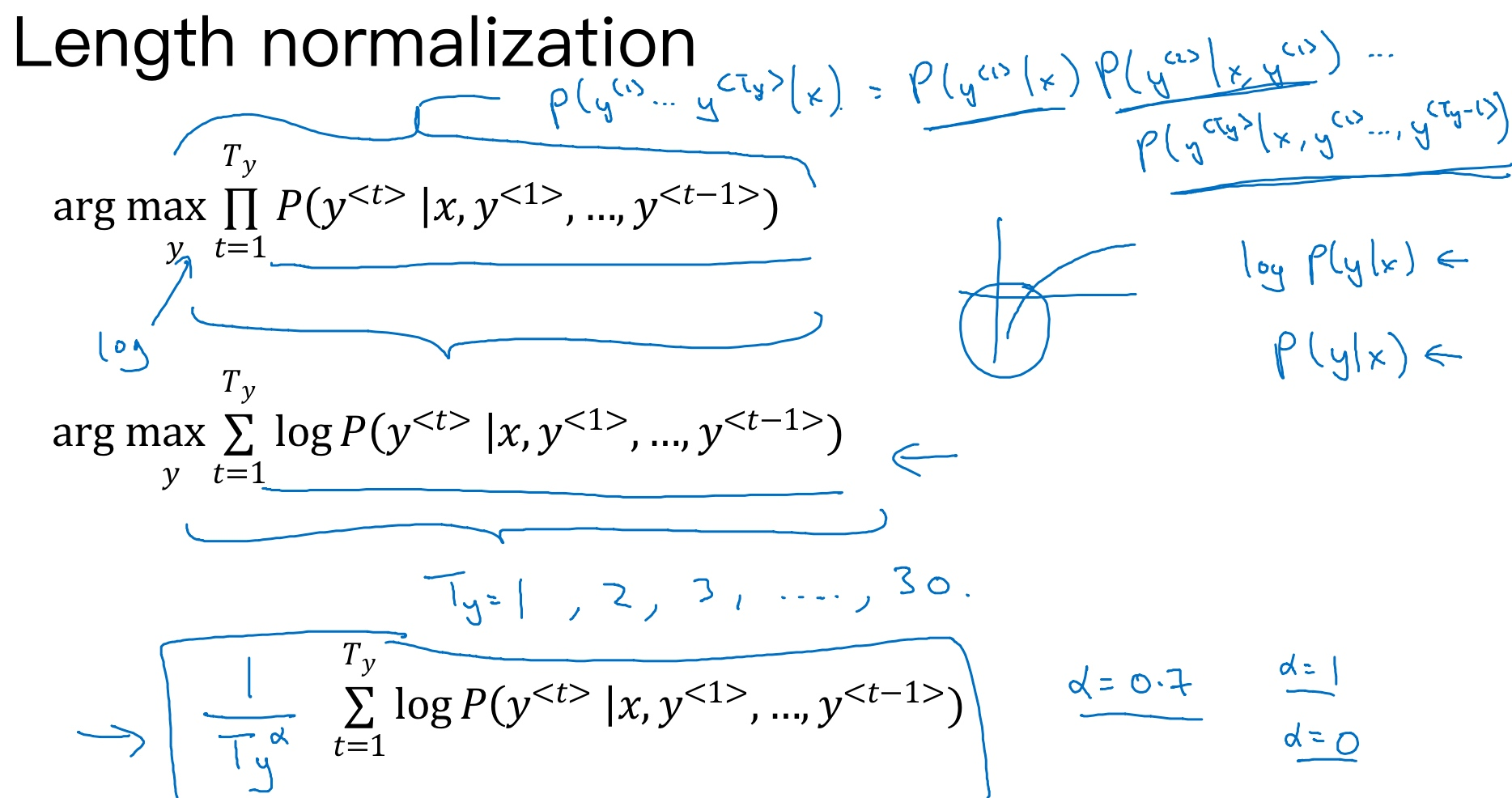Beam search中，最终机器翻译的概率是乘积的形式(集束搜索就是最大化这个概率)：

$arg\ max\prod_{t=1}^{T_y} P(\hat y^{<t>}|x,\hat y^{<1>},\cdots,\hat y^{<t-1>})$

$arg\ max\sum_{t=1}^{T_y} P(\hat y^{<t>}|x,\hat y^{<1>},\cdots,\hat y^{<t-1>})$

log函数式严格单调递增(strictly monotonically increasing)函数, 最大化log P(y|x)的结果应该和最大化 P(y|x) 的结果是一样的. 如果有一个y值能够使log P(y|x)最大化, 那么也一定可以使P(y|x)最大化. 所以实际工作中，总是记录概率的对数和（the sum of logs of the probabilities），而不是概率的乘积（the production of probabilities）

$arg\ max\ \frac{1}{T_y}\sum_{t=1}^{T_y} P(\hat y^{<t>}|x,\hat y^{<1>},\cdots,\hat y^{<t-1>})$

$arg\ max\ \frac{1}{T_y^{\alpha}}\sum_{t=1}^{T_y} P(\hat y^{<t>}|x,\hat y^{<1>},\cdots,\hat y^{<t-1>})$

$\alpha=1$，则完全进行长度归一化；若$\alpha=0$，则不进行长度归一化. 一般令$\alpha=0.7$, 效果不错. $alpha$就是算法另一个超参数（hyper parameter), 需要调整大小来得到最好的结果.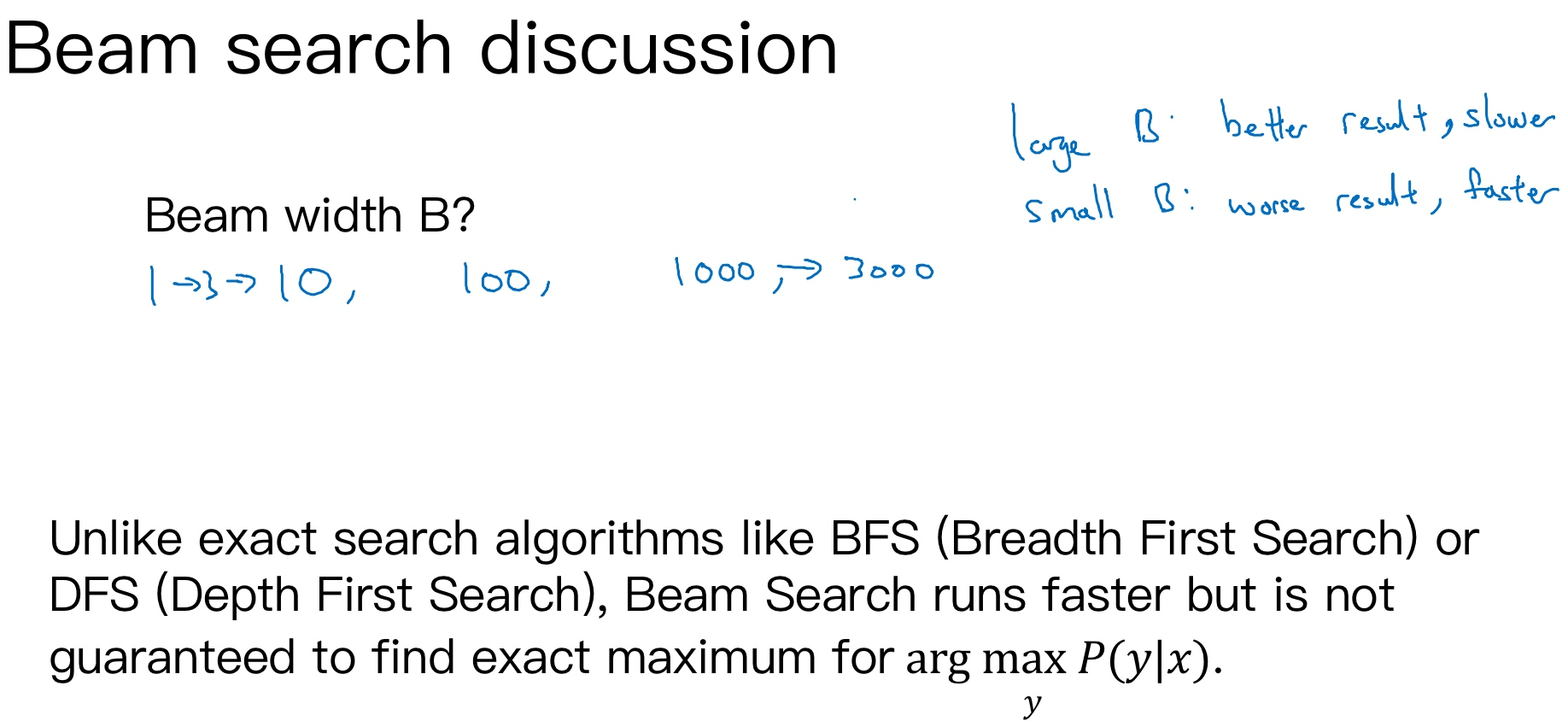• 如果束宽很大，你会考虑很多的可能，你会得到一个更好的结果，但是算法会运行的慢一些，内存占用也会增大，计算起来会慢一点
• 而如果你用小的束宽，结果会没那么好，因为你在算法运行中，保存的选择更少，但是你的算法运行的更快，内存占用也小
• 例子中用了束宽为3，所以会保存3个可能选择，在实践中这个值有点偏小. 在产品中，经常可以看到把束宽设到10

Beam search束搜索算法是一种近似搜索算法（an approximate search algorithm），也被称作启发式搜索算法（a heuristic search algorithm), 它不总是输出可能性最大的句子，它仅记录着B为前3或者10或是100种可能. 实际应用中，如果机器翻译效果不好，需要通过错误分析，判断是RNN模型问题还是beam search算法问题.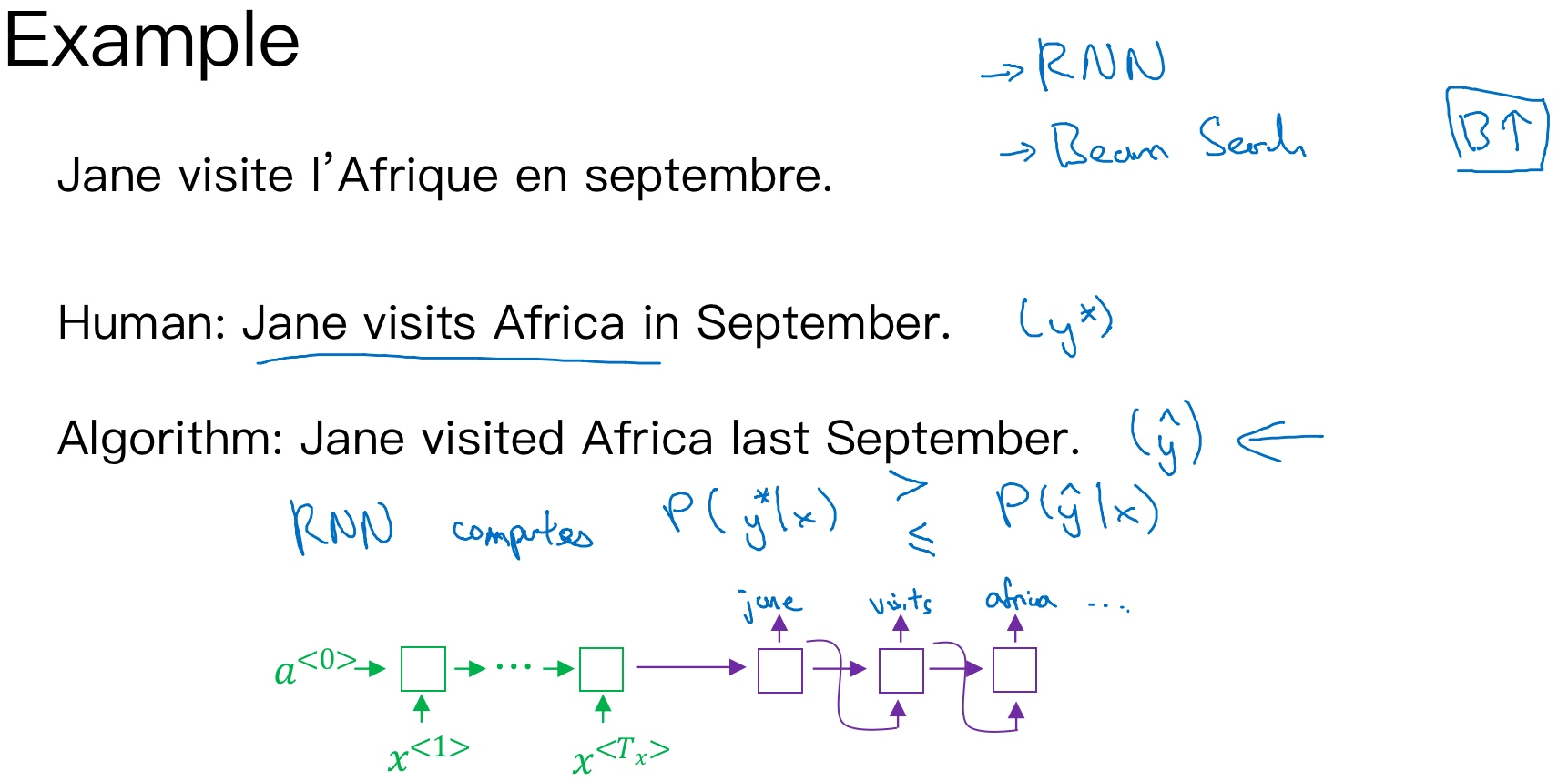• Jane visite l’Afrique en septembre.
• Human: Jane visits Africa in September. $y^*$
• Algorithm: Jane visited Africa last September. $\hat y$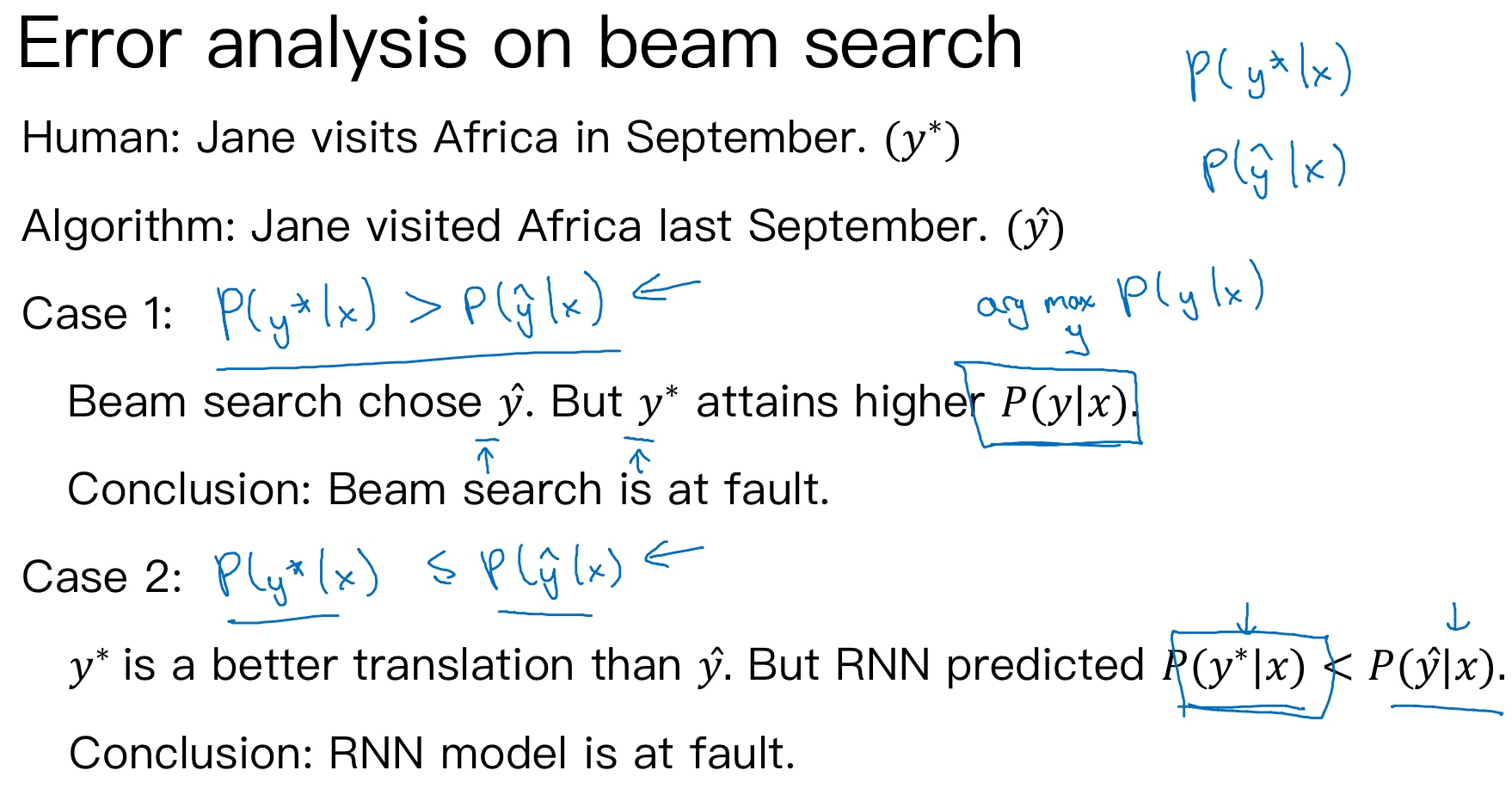• $P(y^*|x) \gt P(\hat y|x)$：Beam search算法有误
• $P(y^*|x) \lt P(\hat y|x)$：RNN模型有误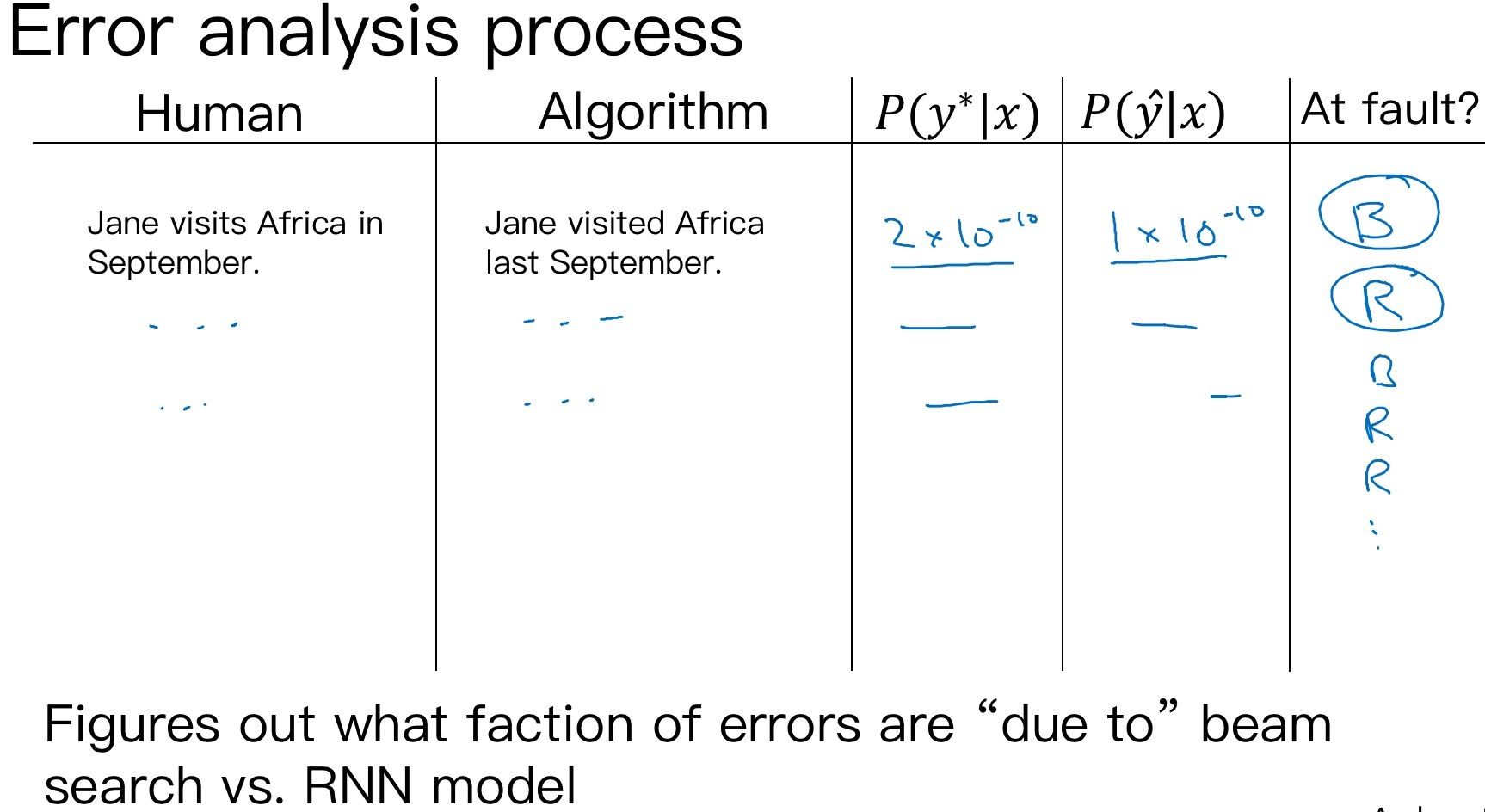## Bleu 得分（选修）（Bleu Score (optional)）

[Papineni, Kishore& Roukos, Salim & Ward, Todd & Zhu, Wei-jing. (2002). BLEU: a Method for Automatic Evaluation of Machine Translation.10.31151073083.1073135.]

• 在戏剧界，侯补演员(understudy)学习资深的演员的角色，这样在必要的时候，他们就能够接替这些资深演员。而BLEU的初衷是相对于请评估员（ask human evaluators），人工评估机器翻译系统（the machine translation system），BLEU得分就相当于一个侯补者，它可以代替人类来评估机器翻译的每一个输出结果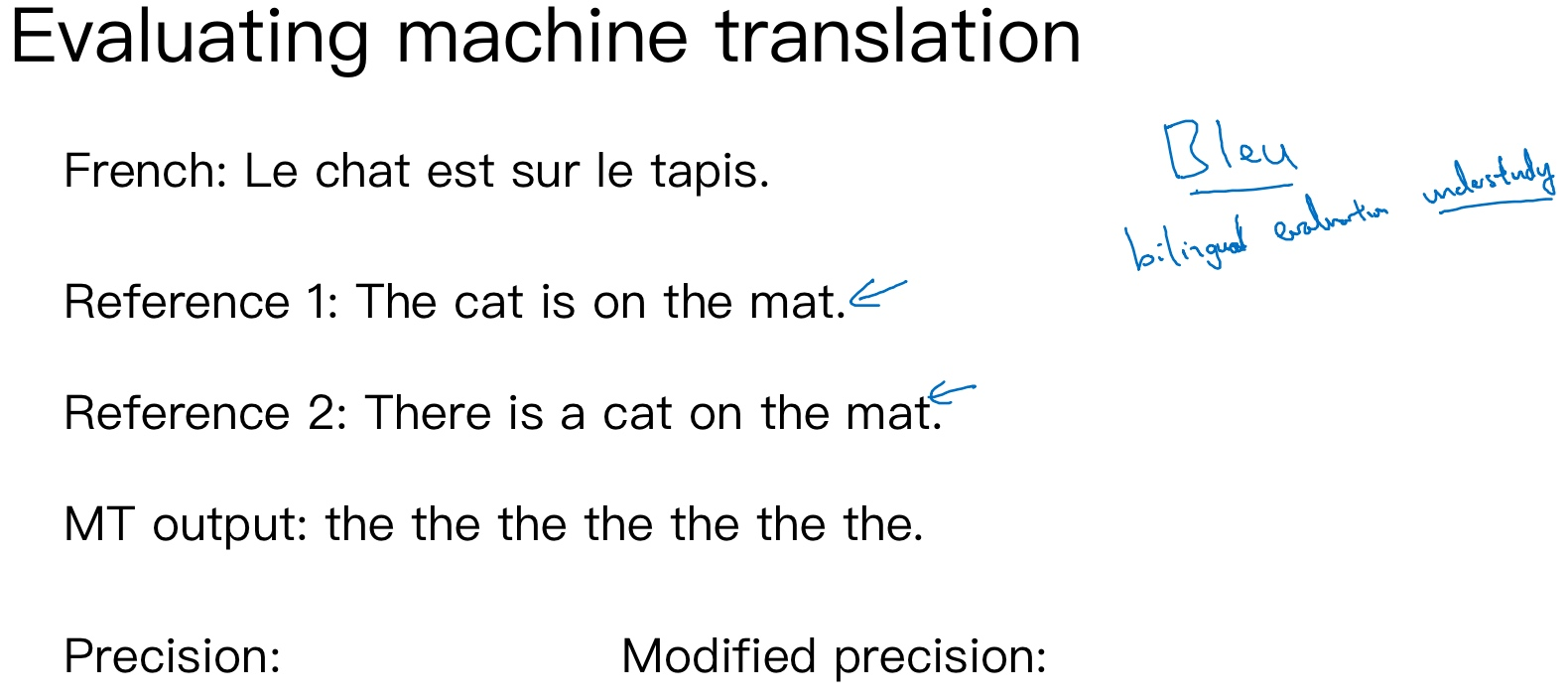• French: Le chat est sur le tapis.
• Reference 1: The cat is on the mat.
• Reference 2: There is a cat on the mat.

• French: Le chat est sur le tapis.
• Reference 1: The cat is on the mat.
• Reference 2: There is a cat on the mat.
• MT output: the the the the the the the.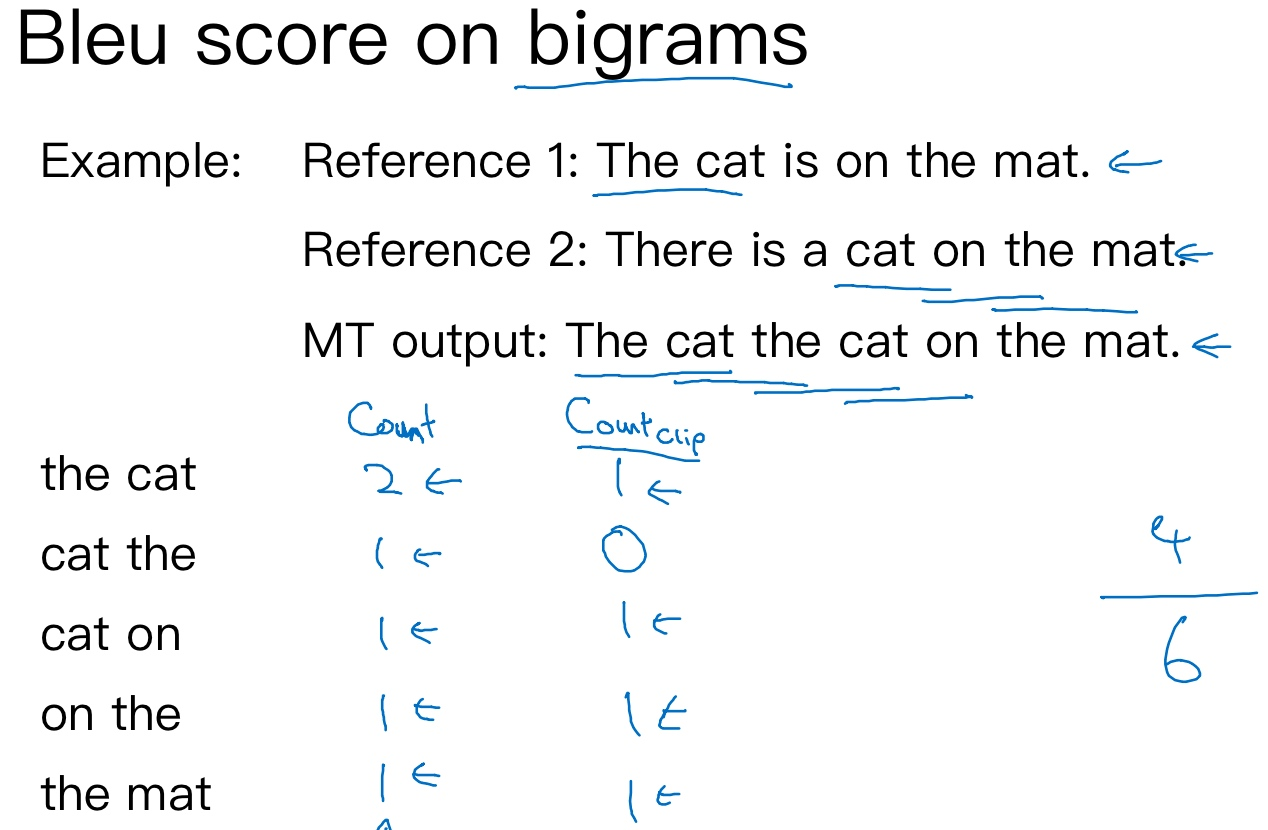• French: Le chat est sur le tapis.
• Reference 1: The cat is on the mat.
• Reference 2: There is a cat on the mat.
• MT output: The cat the cat on the mat.

• the cat: 2
• cat the: 1
• cat on: 1
• on the: 1
• the mat: 1

• the cat: 1
• cat the: 0
• cat on: 1
• on the: 1
• the mat: 1

$\frac{count_{clip}}{count}=\frac{1+0+1+1+1}{2+1+1+1+1}=\frac46=\frac23$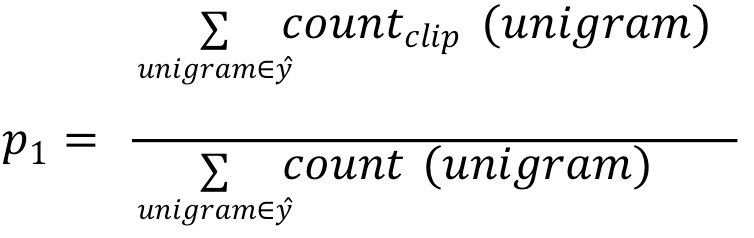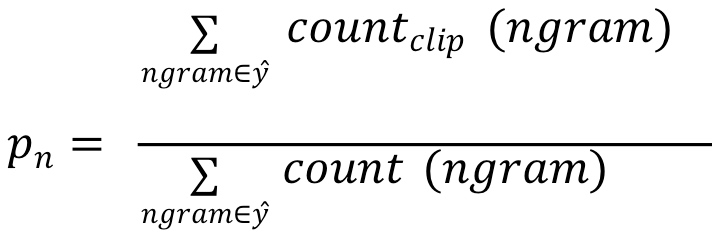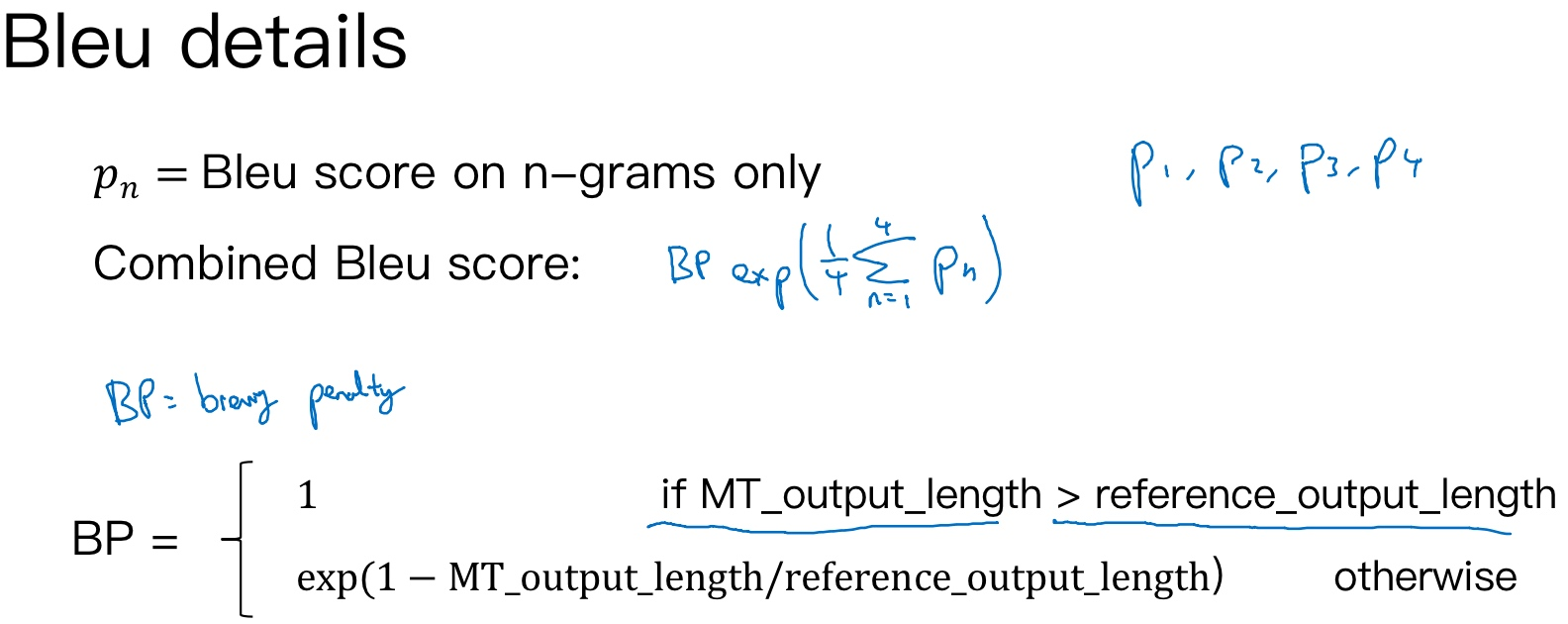$p=\frac1n\sum_{i=1}^np_i$

$p=BP\cdot exp(\frac1n\sum_{i=1}^np_i)$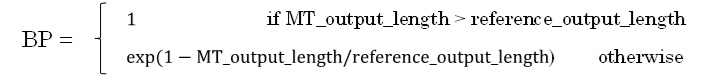## 注意力模型直观理解（Attention Model Intuition）

[Bahdanau et. al., 2014. Neural machine translation by jointly learning to align and translate]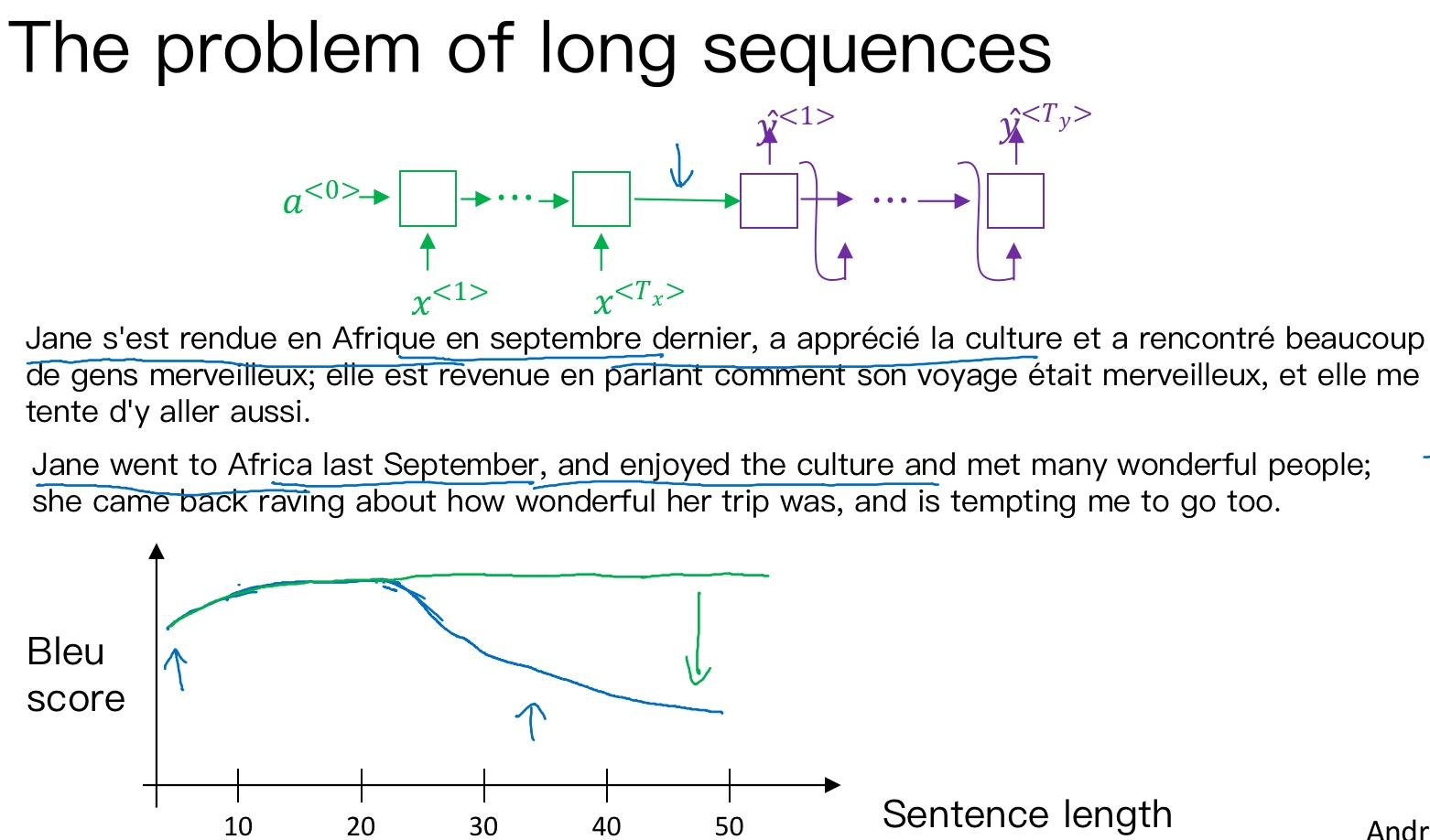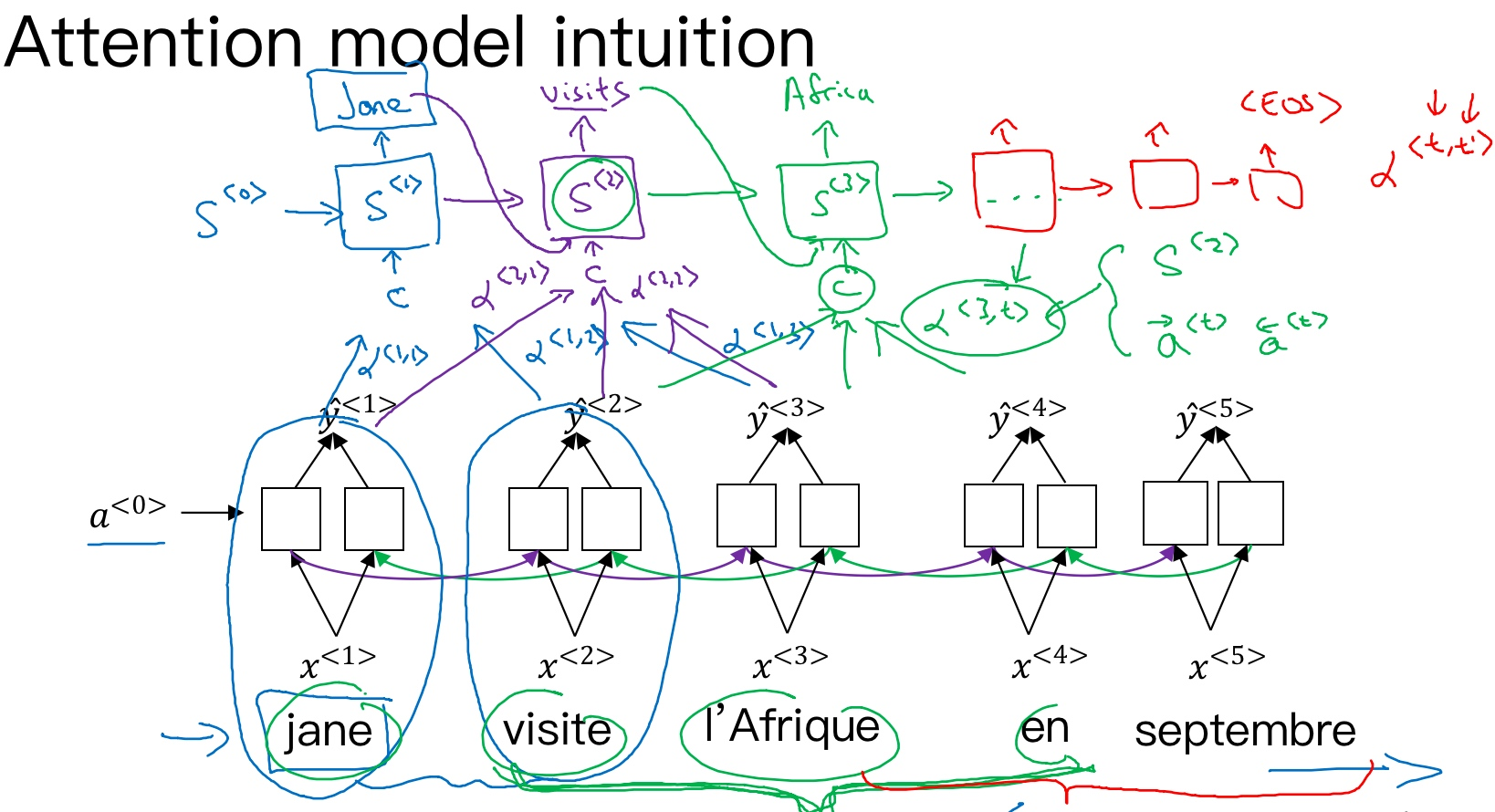Machine translation

like human, looking at part of the sentence at a time (Bleu score(measure the ability neural network to memorize a long sentence) goes up)

first RNN to compute some set of features for each of the input words

Use another RNN to generate English translation

What the Attention Model would be computing is a set of attention weights

When you generating the first word, how much should you be paying attention to first piece of information. Then second ,third and so on; together these will tell us what is exactly the context from the demoter C that we should be paying attention to, and that is input to Second RNN unit to try to generate the first word. then second input derivie from the same way, together with first generated input to generate the second word.

The amounts that this RNN step should be paying attention to the input at time T that depends on the activations of the bidirectional RNN at time T and it will depend on the state from the previous step

RNN marches forward and generate one word at a time until eventually it generates the EOS. Every steps, there are attention weights that tells it when you are trying to generate the English word(t), how much u should be paying attention to the French word(t). And this allows it on every time step to look only maybe within a local window of the French sentence to pay attention to when generating a specific English word.

## 注意力模型（Attention Model）

Attention model中选择双向RNN，可以使用GRU单元或者LSTM. 由于是双向RNN，通过前向和后向的传播，我们可以得到每个时间步的前向和后向的激活值, 用$a^{<t’>}$表示输入句子通过双向RNN(Encoder)得到的每一步的激活值:

$a^{<t’>}=(a^{\rightarrow <t’>},a^{\leftarrow <t’>})$

• $t’$来索引法语句子里面的词

• $\sum_{t’}\alpha^{<1, t^{\prime}>}=1$

• $C^{<t>}=\sum_{t’}\alpha^{<t, t^{\prime}>} \cdot a^{<t′>}$
• 即: Decoder的输入变成不同的上下文C, 且不同上下文C是由输入单词的权重和激活值相乘得来的.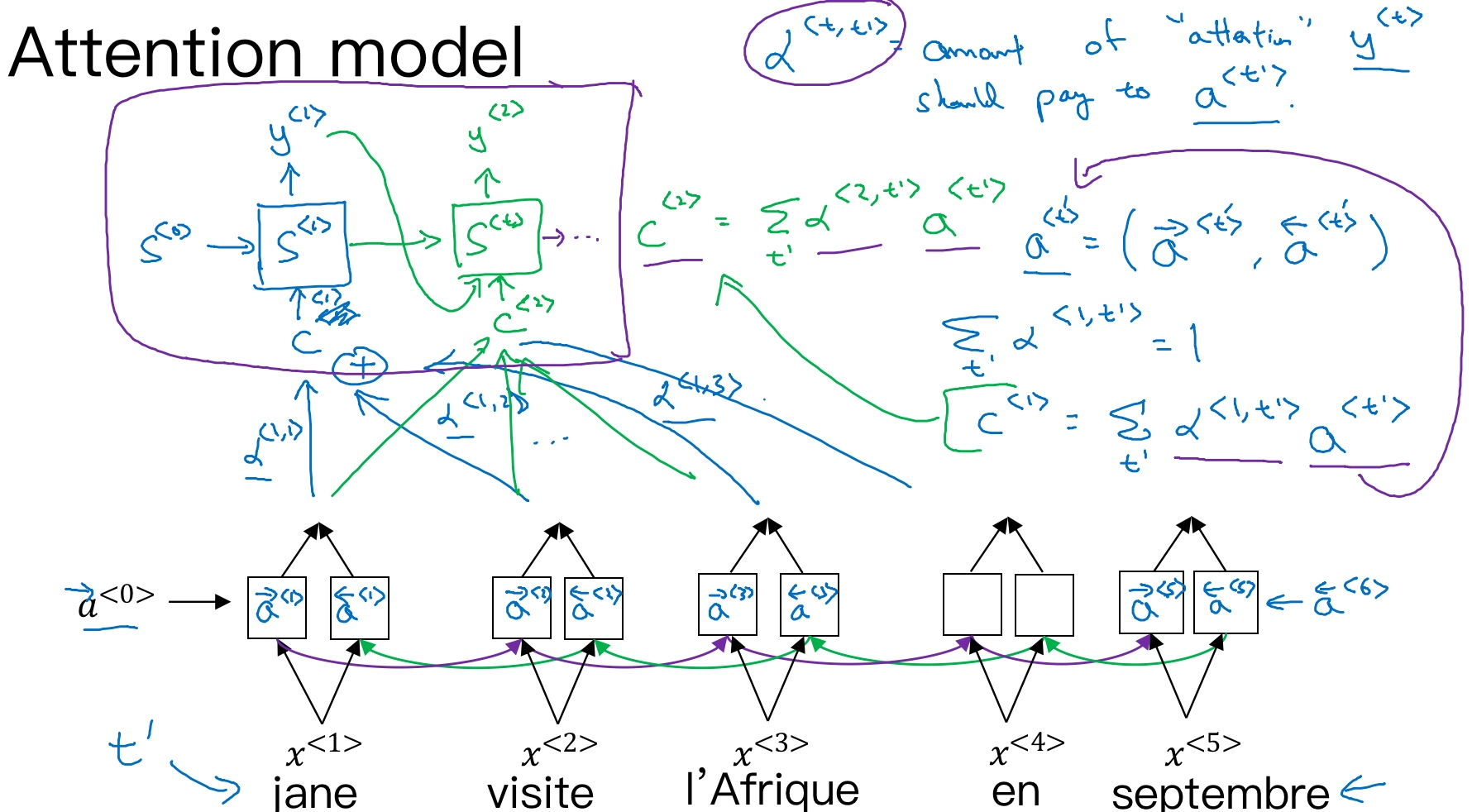$\alpha^{<t, t′>}=\frac{exp(e^{<t, t′>})}{\sum_{t’=1}^{Tx}exp(e^{<t, t′>})}$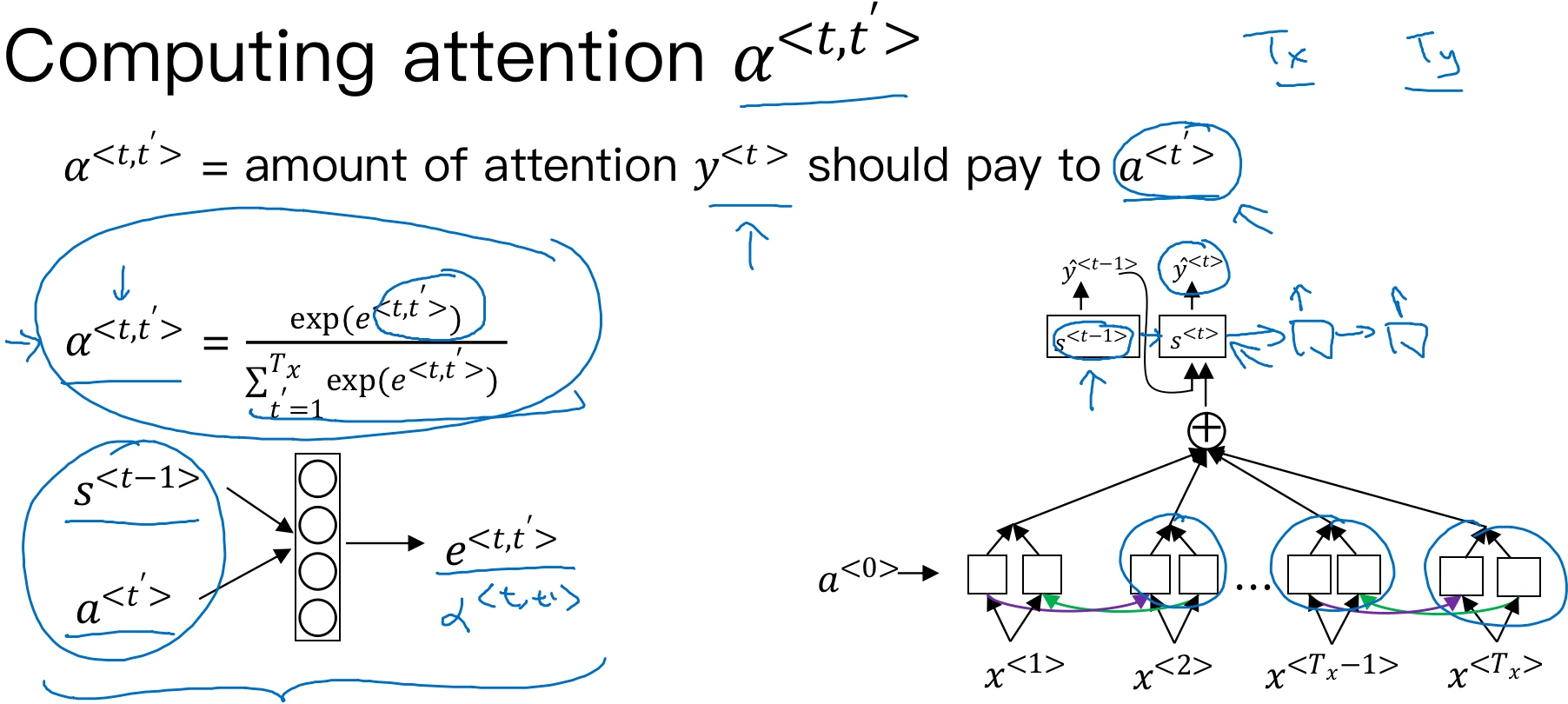Attention model的一个缺点是其计算量较大 (具有$O(T_xT_y)$的复杂度)，若输入句子长度为$T_x$, 输出句子长度为$T_y$, 则计算时间约为$T_x*T_y$. 但是，其性能提升很多，输入和输出的句子一般不会太长，这样的复杂度是可以接受的.

Attention model在图像捕捉方面也有应用.

Attention model能有效处理很多机器翻译问题，例如:

• 将不标准的时间格式转换为统一的时间格式
• 将注意力权重可视化：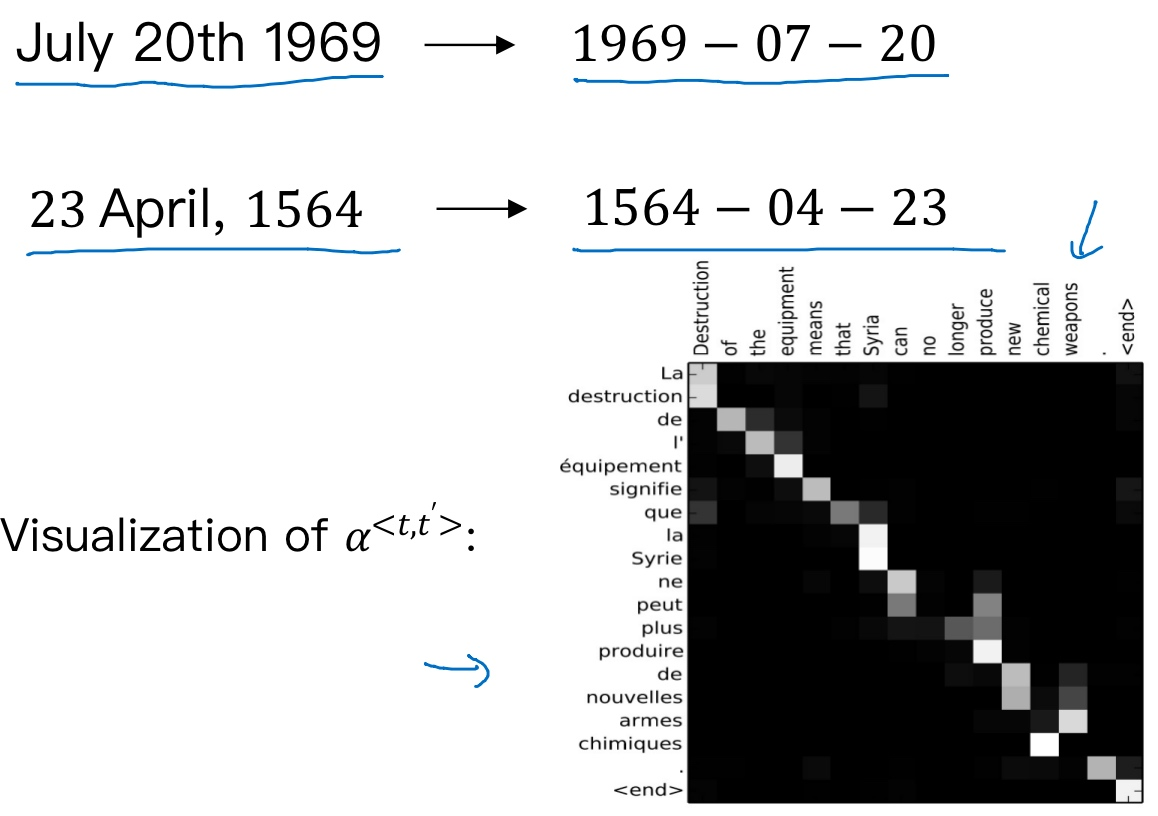### attention小结

x,y就是输入输出; h就是encoder的隐状态(激活值); h’就是decoder的隐状态(激活值); c就是上下文context. Encoder每个时刻的输入是上一个时刻的隐状态(激活值)和输入(vector)，然后会有一个输出(vector)和新的隐状态(激活值). 这个新的隐状态(激活值)会作为下一个时刻的输入隐状态(激活值). 每个时刻都有一个输出(vector)，对于seq2seq模型来说，通常只保留最后一个时刻的隐状态(激活值)，认为它编码了整个句子的语义 (Attention机制会用到Encoder每个时刻的输出(vector)). 最后, Encoder的输出是一个vector和一个隐状态(激活值). 而得到c有多种方式, 最简单的方法就是把Encoder的最后一个隐状态(激活值)赋值给c，还可以对最后的隐状态(激活值)做一个变换得到c，也可以对所有的隐状态(激活值)做变换. 最后c作为Decoder的初始隐状态.

• $c_i = \sum_{j=1}^{T_x} \alpha_{ij}h_j$
• $\alpha_{ij} = \frac{exp(e_{ij})}{\sum_{k=1}^{T_x} exp(e_{ik})}$
• $e_{ij} = score(h'_{i-1},h_j)$h’就是ht, 就是decoder的隐藏态(激活值); h就是hs, 就是encoder的隐藏态(激活值).## 语音识别（Speech recognition）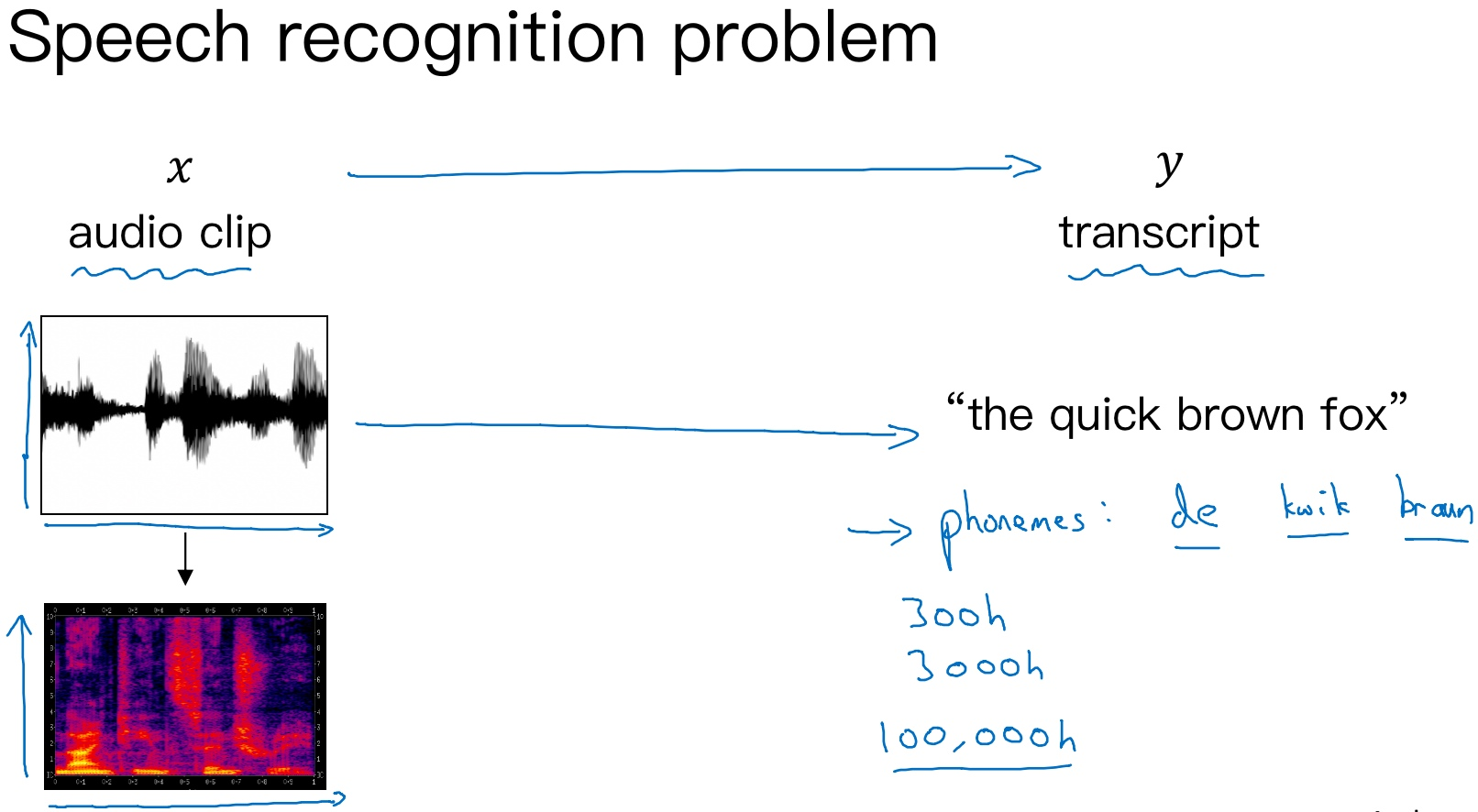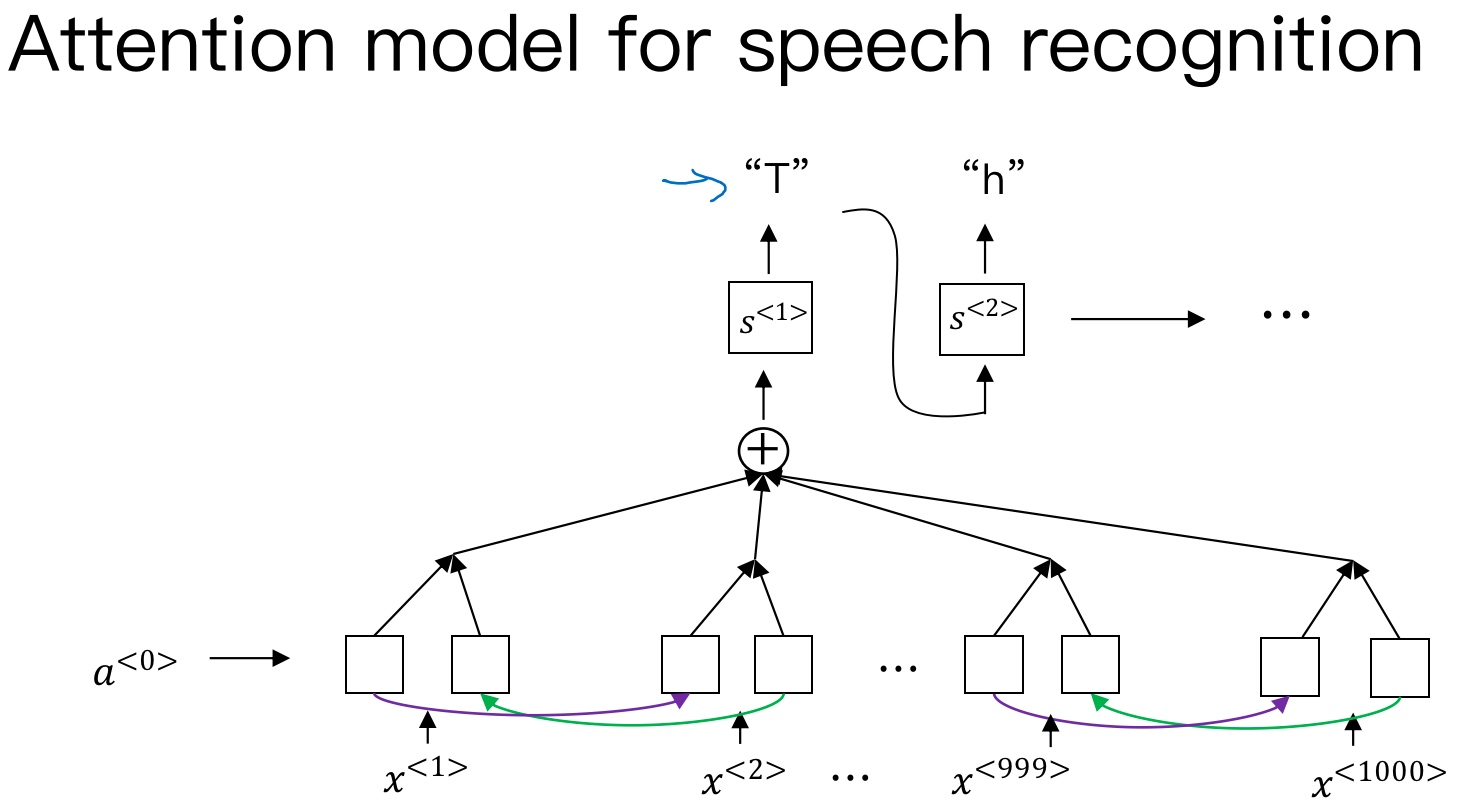CTC 损失函数的语音识别:

[Graves et al., 2006. Connectionist Temporal Classification: Labeling unsegmented sequence data with recurrent neural networks]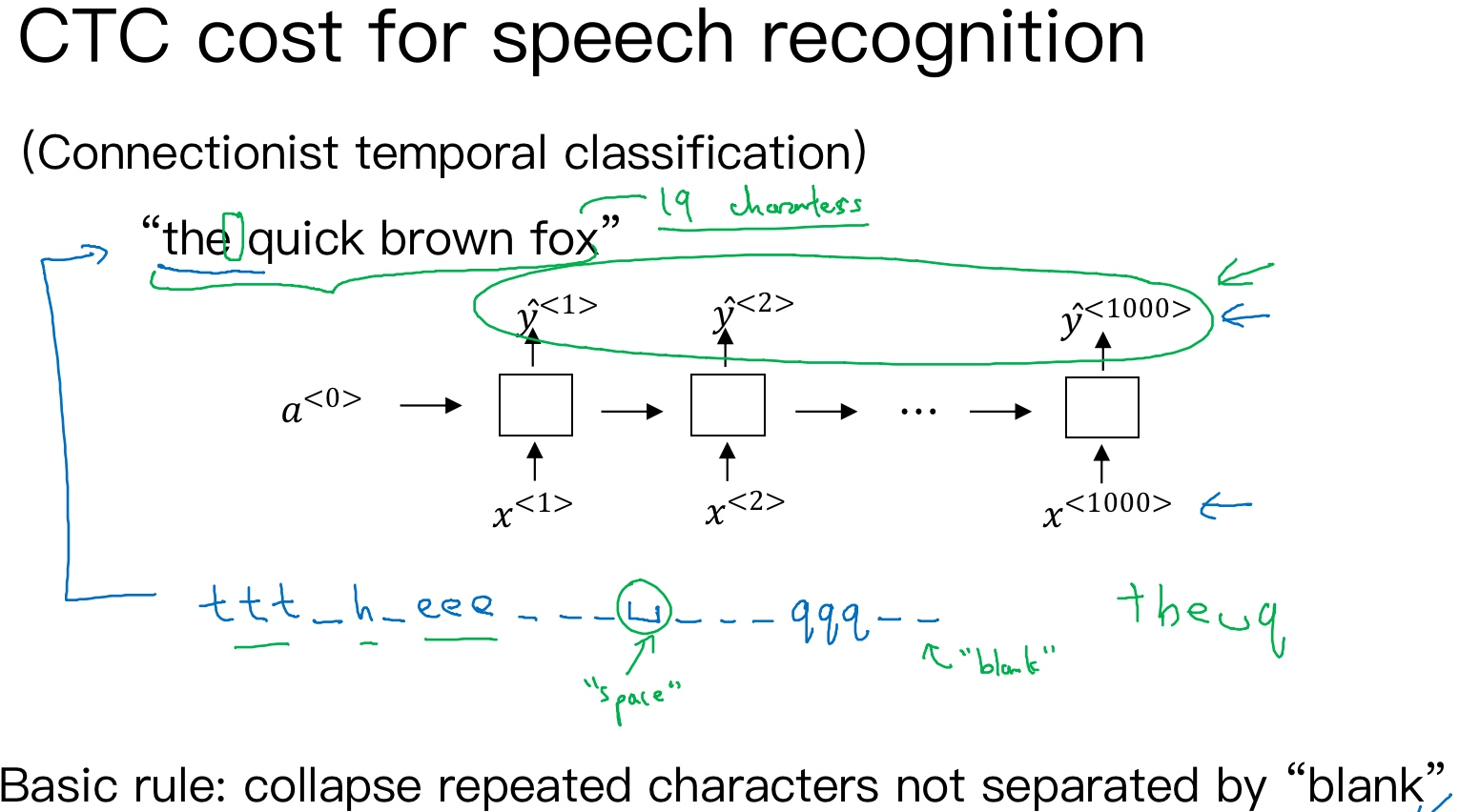## 触发字检测（Trigger Word Detection）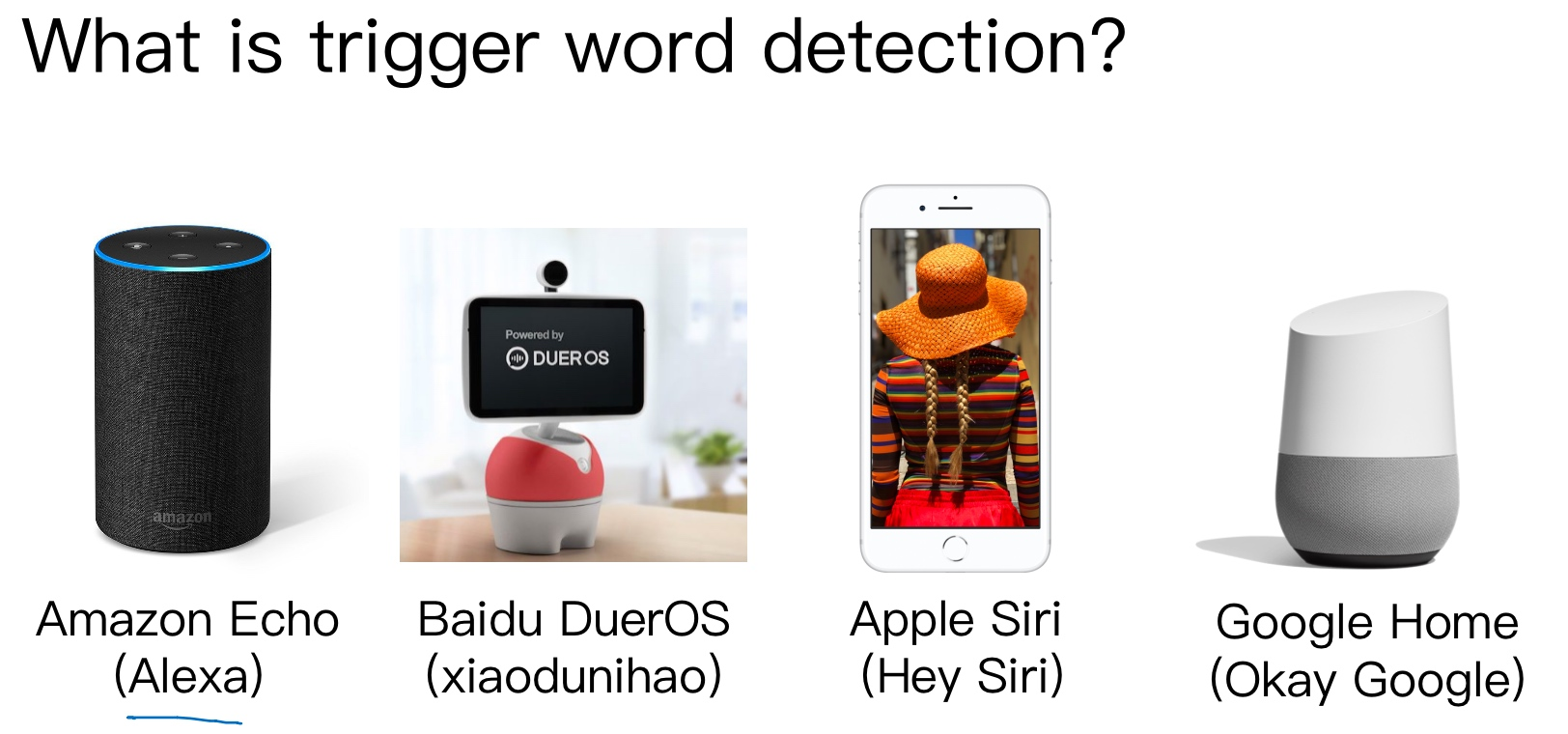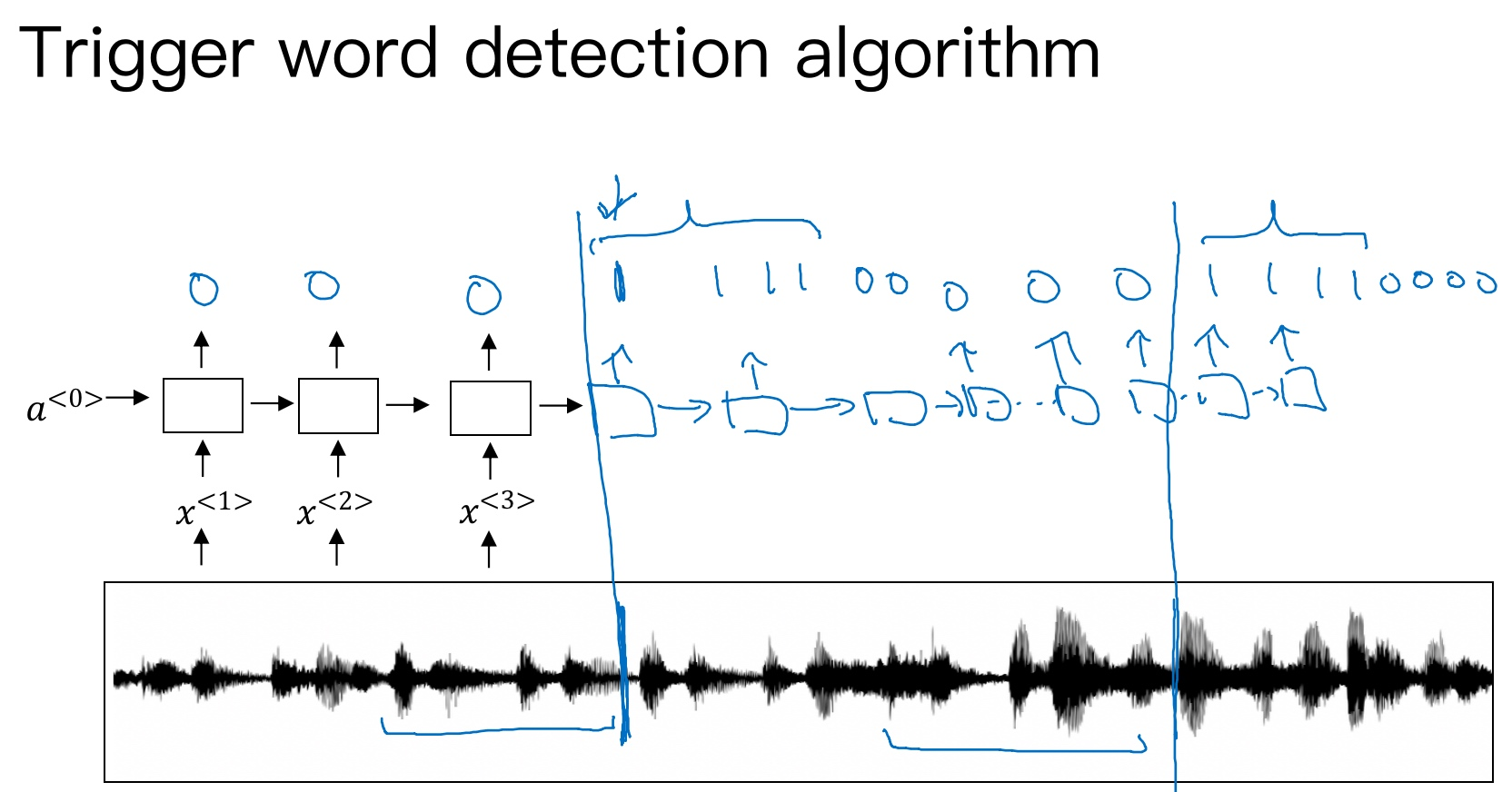ref: